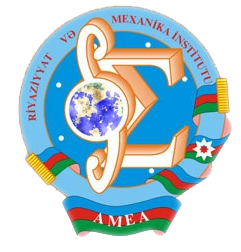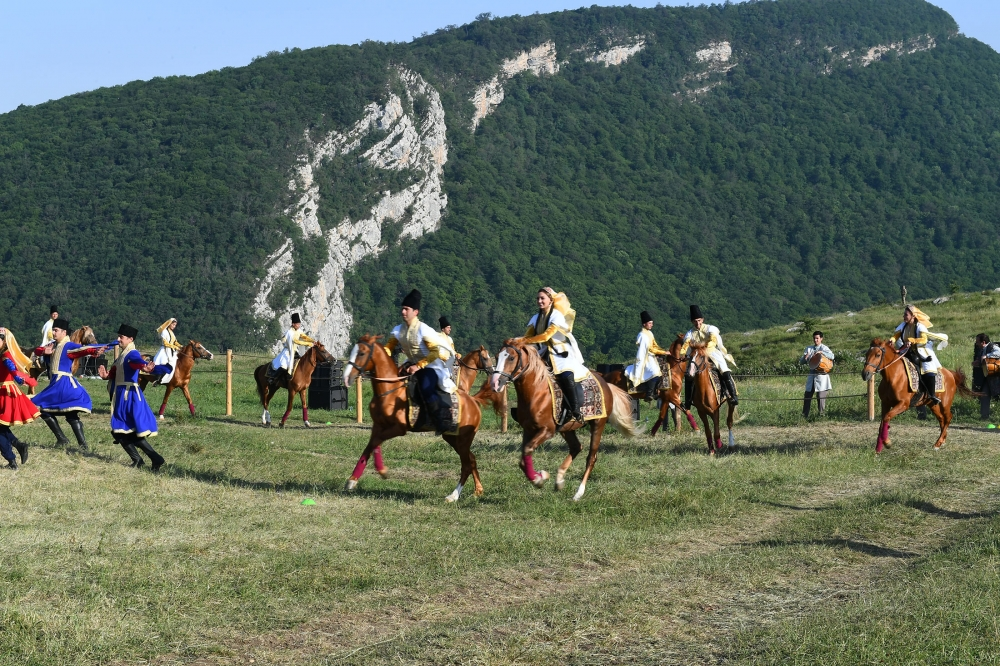Riyaziyyat və Mexanika İnstitutu

## Qarabağ xəbərləri### Şuşada Heydər Əliyev Fondunun təşkilatçılığı ilə “Musiqi irsi və Qarabağ atları Cıdır düzündə” adlı kompozisiya təqdim olunub## Transactions 2005/1/XXV

 Year: 2005 Volume: 1 Number: XXV MATHEMATICS Abbasov A.Sh. Abstract [PDF] On correct solvability of some boundary value problems for operator-differential equations of odd order [PDF] 3 Akhmedov M.A. Abstract [PDF] On compactness of imbedding for one class of weight anisotropic pseudo-normalized spaces [PDF] 11 Aliyev R.T. Abstract [PDF] On ergodic characteristics of the semi-markovian random walk with negative drift positive jumps and two barriers [PDF] 19 Amanov R.A. Abstract [PDF] Regularity of boundary point for non-uniformly degenerating second order elliptic equations [PDF] 23 Aslanova N.M. Abstract [PDF] Stability of reconstruction of the Sturm-Liouville operator with matrix coefficients on scattering data [PDF] 33 Babaev M-B.A. Abstract [PDF] Finding the extremals of the optimal approximation on the set different from parallelepiped [PDF] 43 Gadjiev T.S., Gasimova E.R. Abstract [PDF] On smoothness of solution of the first boundary value problem for second order degenerate elliptic-parabolic equations [PDF] 53 Guseynov F.B. Abstract [PDF] Asymptotics as t-t +oo of solution of Cauchy problem for Sobolev-Galpern time derivative of the first order equation [PDF] 67 Hasanova S.H. Abstract [PDF] On a uniqueness of strong solution of Dirichlet problem for second order quasilinear parabolic equations [PDF] 77 Heydarov A.H. Abstract [PDF] One-dimensional Schrodinger operator with &’-interaction [PDF] 83 Ismailov V.E. Abstract [PDF] On two-sided exact estimates for the best approximation by sums vW + ^Jf) [PDF] 89 Khaniyev T.A. Abstract [PDF] About moments of generalized renewal process [PDF] 95 Mamedova V.A. Abstract [PDF] On removable sets of solutions of boundary value problems for elliptic equations of the second order [PDF] 101 Mammadov M.A. Abstract [PDF] Invariant determination of ^-operators [PDF] 107 Mirzoyev V.S. Abstract [PDF] On closure of algebra of piecewise-continuous functions[PDF] 113 Nabiev I.M., Osmanova J.A. Abstract [PDF] Solution of the inverse problem for a system of equations on a segment[PDF] 119 Panakhov E.S. Abstract [PDF] On inverse problem for singular Sturm-Liouvillc operator from two spectra[PDF] 125 Rzayev K.U. Abstract [PDF] On uniqueness of solutions of Cauchy type problem for uncountable normal systems with first order partial derivatives [PDF] 135 Salimov M.Yu. Abstract [PDF] On correct solvability of a second order boundary-value problem for one class of operator-differential equation on finite segment [PDF] 141 Salmanov V.F. Abstract [PDF] Completeness and minimality of one system of exponents in space of piecewise continuous functions [PDF] 147 MECHANICS Aliyev G.A. Abstract [PDF] Pulsating flow of bubble liquid in a visco-elastic tube non-homogeneous in length [PDF] 153 Mamedov Kh.B. Abstract [PDF] Refined methods for investigation of deflected mode of adjoint structural elements of shell [PDF] 161 Namazov S.N. Abstract [PDF] Some problems of fatigue strength characteristics of sintered steel[PDF] 167 Ramazanov T.G., Mamedzade R.A. Abstract [PDF] Nonlocal axisymmetric fluid filtration in viscoelastic strata[PDF] 171 APPLIED PROBLEMS OF MATHEMATICS AND MECHANICS Akhmedov T.M. Abstract [PDF] The role of fractional operators in electrodynamics[PDF] 181 Aliev G.F. Abstract [PDF] The approximation and convergence of boundary conditions of a fourth order equation [PDF] 195 Aliev F.A., Niftiev A.A., Akhundov H.S., Aliev V.F. Abstract [PDF] Mathematical simulation and optimal distribution of resources in industrial units [PDF] 201 Sadygov M.A. Abstract [PDF] On bisnbdifferentials of biconvex operators [PDF] 209 Tagiyev R.K. Abstract [PDF] On optimal control for processes descrilted by quasilinear elliptic equations[PDF] 217
Azərbaycanda COVID-19 ilə bağlı statistika
• Virusa yoluxan

598503

• Sağalan

567385

• Yeni yoluxan

0

• Aktiv xəstə

23114

• Ölüm halı

8004

• Test edilib

5,621,021